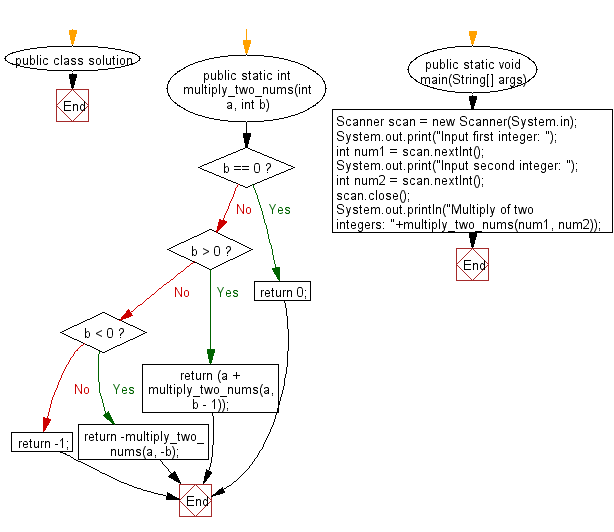﻿ Java Math Exercises: Multiply two integers without using multiplication, division, bitwise operators, and loops - w3resource# Java Math Exercises: Multiply two integers without using multiplication, division, bitwise operators, and loops

## Java Math Exercises: Exercise-15 with Solution

Write a Java program to multiply two integers without using multiplication, division, bitwise operators, and loops.

Sample Solution:

Java Code:

``````import java.util.*;
public class solution {
public static int multiply_two_nums(int a, int b) {

/* 0 multiplied with anything gives 0 */
if (b == 0)
return 0;

if (b > 0)
return (a + multiply_two_nums(a, b - 1));

if (b < 0)
return -multiply_two_nums(a, -b);

return -1;
}

public static void main(String[] args) {
Scanner scan = new Scanner(System.in);
System.out.print("Input first integer: ");
int num1 = scan.nextInt();
System.out.print("Input second integer: ");
int num2 = scan.nextInt();
scan.close();
System.out.println("Multiply of two integers: "+multiply_two_nums(num1, num2));
}
}
``````

Sample Output:

``` Input first integer:  5
Input second integer:  25
Multiply of two integers: 125
```

Flowchart:Java Code Editor:

What is the difficulty level of this exercise?

﻿

## Java: Tips of the Day

Parsing dates:

```import java.io.*;
import java.util.*;
import java.text.*;

String s = "2001/09/23 14:39";

SimpleDateFormat formatter = new SimpleDateFormat ("yyyy/MM/dd H:mm");
Date d = formatter.parse(s, new ParsePosition(0));
```